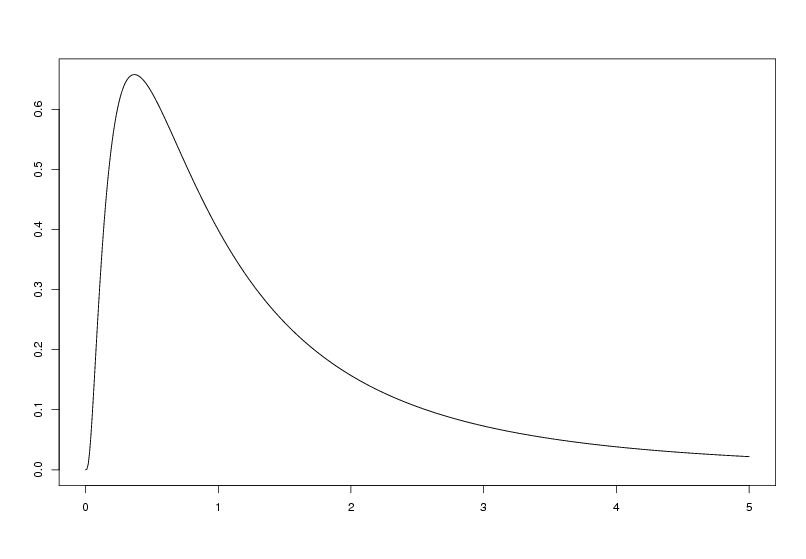0
Correct
0
Incorrect
3
Total

## Question 1 of 3

An operation in a production company requires a certain training for new employees. A new training program was proposed and compared to the standard program. Nine new employees were trained using the standard method and other nine new employees using the new method. The time it took for the eighteen employees to finish the operation was then registered.

The measurements are (in minutes):

New method 35 31 29 25 34 40 27 32 31
Standard method 32 37 35 28 41 44 35 31 34

If the measurements for the new program can be assumed to be normally distributed, $N(\mu,\sigma^2)$, the critical value for the usual test of the hypotheses $H_0$: $\mu=30$ vs. $H_1$: $\mu\not= 30$ using $\alpha=5\%$ (based on the 9 measurements) is:

## Question 2 of 3

Out of 170 winners in as many dog races, 35 dogs started on the inner line.

The number of winners out of the 170 that did not start at the inner lane could have the following distribution:

## Question 3 of 3

In the following figure a density function is shown:Which of the following probability distributions is the only one that could be described by the the above figure?